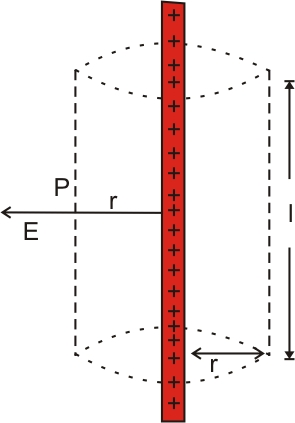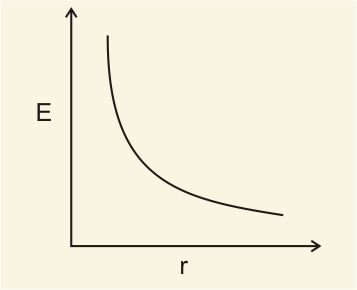# (a) Use Gauss’ law to derive the expression for the electric field due to a straight uniformly charged infinite line of charge density (b) Draw a graph to show the variation of E with perpendicular distance r from the line of charge. (c) Find the work done in bringing a charge q from perpendicular distance

a) Electric field due to an infinitely long straight charged wireConsider infinitely long straight charged wire of linear charge density
For calculating electric field consider an imaginary cylindrical Gaussian Surface of radius r and length l.
Here the field is radial everywhere, so flux through the two ends of cylinder is Zero.
At the Gaussian cylindrical surface, the electric field E is normal to the surface at every point. The magnitude of E depends only on radius 'r', so it is constant.
Therefore flux through Gaussian surface

According to Gauss's law , flux

Here, total charge enclosed = linear charge density length

Therefore flux
Using equation (1) and (2)
That is ,

The vector notation is

where is the radial unit vector normal to the line charge
b)c) work done to move the charge 'q' through a small displacement 'dr'

We know ,
where E = electric field
Therefore
Substitute the value of E

work done in moving the charge from r1 to r2 is

## Related Chapters

### Preparation Products

##### Knockout KCET 2021

An exhaustive E-learning program for the complete preparation of KCET exam..

₹ 4999/- ₹ 2999/-
##### Knockout KCET JEE Main 2021

It is an exhaustive preparation module made exclusively for cracking JEE & KCET.

₹ 27999/- ₹ 16999/-
##### Knockout NEET Sept 2020

An exhaustive E-learning program for the complete preparation of NEET..

₹ 15999/- ₹ 6999/-
##### Rank Booster NEET 2020

This course will help student to be better prepared and study in the right direction for NEET..

₹ 9999/- ₹ 4999/-